﻿ Deciding if a Number is a Whole Number, Integer, etc.
DECIDING IF A NUMBER IS A WHOLE NUMBER, INTEGER, etc.

Numbers have lots of different names!
Even though $\;4\;$ and $\;\frac{8}{2}\;$ and $\;5-1\;$ and $\;4.00\;$ and $\;2^2\;$ all look different,
they are all representations for the same number.

Although numbers come in lots of different sizes, and have lots of different names, here's the good news:
all these numbers live on the ‘line’ shown below.
This is called a real number line, and is the subject of this section.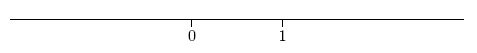A real number line is determined by three pieces of information:

• a (straight) line;
(Even though this line may have any orientation, the following discussion assumes that the line is horizontal.)
• a point on the line, usually labeled as the number $\;0\;$ (zero); and
• a second point on the line, to the right of the first point, usually labeled as the number $\;1\;$ (one).
Sometimes, an arrow is put at the right end of a number line, to show that this is the ‘positive’ direction;
that is, the numbers increase as you move to the right.Sometimes, arrows are put at both ends, to suggest that the line extends forever in both directions.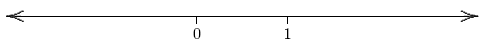Sometimes, there are no arrows at all: this is the simplest representation, and is the one that will be used most often on this website.

With choices made for $\;0\;$ and $\;1\;$, the locations of all other numbers are uniquely determined .
For example, the locations of these three numbers are shown below:

• $\;-1\;$ (negative one)
• $\;\frac12\;$ (one-half)
• $\;3.5\;$ (‘three point five’ or ‘three and five-tenths’)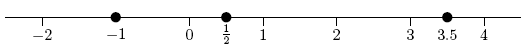Even though two different numbers are required to determine where all the other numbers live, people occasionally get lazy.

If there's only a single number that is currently of interest, then a ‘number line’ may be drawn showing only that particular number.
For example, all numbers to the right of $\;2\;$ might be illustrated like this: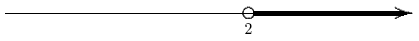(The hollow dot at $\;2\;$ indicates that $\;2\;$ is not to be included.)

A number line provides us with a picture of a collection of numbers referred to as the real numbers.
It is a conceptually perfect picture, in the following sense:

• every point on the line corresponds to a real number; and
• every real number corresponds to a point on the line.
Since there is this ‘pairing’ of real numbers and points on the line, people tend to use the words ‘number’ and ‘point’ interchangeably.
So will this author.

The symbol $\;\mathbb{R}\;$ (‘blackboard bold R’) is used to denote the set of real numbers.

The numbers to the right of zero are called the positive real numbers;
the numbers to the left of zero are the negative real numbers.

The number zero is not positive (since it doesn't lie to the right of zero), and not negative (since it doesn't lie to the left of zero).
Zero is the only real number with this ‘neutral’ status; every other real number is either positive or negative.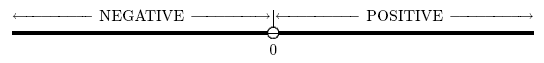Which real numbers are not negative?
Zero isn't negative.
Also, the positive numbers are not negative.
These numbers—zero, together with all real numbers to the right of zero—are called the nonnegative real numbers, and are shaded below.
The solid (filled-in) dot at $\;0\;$ indicates that $\;0\;$ is being included; the arrow to the right indicates that the shading is to continue for all numbers to the right of zero.A nonzero real number is one that is not zero; the nonzero real numbers are shaded below.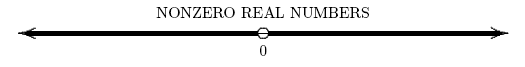There are some important subcollections of the real numbers that are given special names.
The whole numbers are the subcollection containing:   $0$, $1$, $2$, $3$, $\dots$

The three lower dots ‘ $\;\ldots\;$’ indicate that the established pattern is to be repeated ad infinitum
(pronounced odd in-fi-NIGHT-um or add in-fi-NIGHT-um; means forever and ever).

Thus, $\;127\;$ is a whole number, but $\;\frac12\;$ isn't.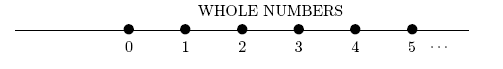Be careful! Numbers have lots of different names. Either a number is a whole number, or it isn't.
The particular name being used doesn't matter.
For example, the number $\;3\;$ is a whole number.
The number $\;3\;$ has many names, like $\;\frac62\;$ and $\;2.9+0.1\;$.
So, $\;\frac62\;$ is a whole number and $\;2.9+0.1\;$ is a whole number.
Don't let the name being used lead you astray!

Consecutive whole numbers are whole numbers that follow one after the other, without gaps.
The phrase can refer to just two numbers, or more than two.
Thus, $\;2\;$ and $\;3\;$ are consecutive whole numbers;
$\;5\;$, $\;6\;$, and $\;7\;$ are consecutive whole numbers;
$\;2\;$ and $\;5\;$ are not consecutive whole numbers.

Notice how sparse the whole numbers are, as they sit in the collection of real numbers!
Between any two consecutive whole numbers are an infinite (IN-fi-nit) number of real numbers, that are NOT whole numbers.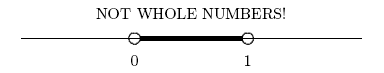There are two different concepts frequently used to compare numbers:

• SIZE:   the size of a number refers to its distance from zero.
The words ‘bigger’ and ‘smaller’ are used to talk about size.
• ORDER:   there is a natural left/right ordering on the number line.
Given any two numbers, either they are equal, or one lies to the right of the other.
The words ‘greater than’ and ‘less than’ are used to talk about order.

The size of real numbers is discussed next.
Order will be discussed in the future section I Live Two Blocks West Of You.

Roughly, a number is big or large if it is far from zero:Note that big/large numbers can be positive (like $1{,}000{,}000$; one million) or negative (like $-1{,}000{,}000$; negative one million).

Roughly, a number is small if it is close to zero:Note that small numbers can be positive (like $\frac{1}{1000}$; one thousandth) or negative (like $-\frac{1}{1000}$; negative one thousandth).
Usually, ‘small’ means close to zero, but not equal to zero.

Numbers like $\;2\;$ and $\;-2\;$ are called opposites:
they have the same distance from zero, but are on opposite sides of zero.
The opposite of a positive number is a negative number.
The opposite of a negative number is a positive number.
The opposite of zero is zero: zero is the only real number that is its own opposite.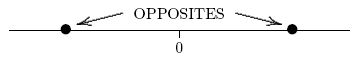Whenever you add a number to its opposite, you get zero as a result:

$$3 + (-3) = 0$$ $$(-2) + 2 = 0$$ $$5.1 + (-5.1) = 0$$ $$0 + 0 = 0$$

The number $\;-3\;$ can be read as either negative three or the opposite of three.
Many people favor negative three, because it's faster. However, both ways are correct.
Try not to read it as minus three, because the word minus is reserved for the operation of subtraction.

Similarly, $\;-x\;$ can be read as either negative ex or the opposite of ex.
Here, the letter $\;x\;$ is being used to represent a number:
such use of letters to represent numbers is discussed in the section Holding This, Holding That.
We'll see in this future section that it is preferable for beginning students of mathematics to read $\;-x\;$ as the opposite of $\;x\;$ .

When we take the whole numbers, and throw in their opposites,
then we get the important subcollection of the real numbers that is called the integers (IN-teh-jers).
Thus, the integers are the subcollection: $$\ldots, -3, -2, -1, 0, 1, 2, 3, \ldots$$

The symbol $\;\mathbb{Z}\;$ is used to denote the set of integers.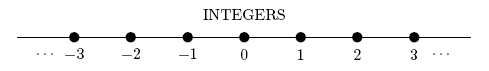One important property of the real numbers is that they are dense;
that is, between every two different real numbers (no matter how close they are), there is another real number.
This is often called the density property of the real numbers.
Indeed, between every two different real numbers, there are an infinite number of real numbers!If two numbers live at the same place on a real number line, then we say that they are equal.
And, if two numbers are equal, this means that they live at the same place on a real number line.

The mathematical sentence ‘$\;a = b\;$’ is read as ‘$\,a\,$ equals $\,b\,$’ or ‘$\,a\,$ is equal to $\,b\,$’.
This sentence is true if $\,a\,$ and $\,b\,$ live at the same place on a real number line; otherwise, it's false.
Note that if the sentence ‘$\;a = b\;$’ is true, then you're being told that $\,a\,$ and $\,b\,$ are just different names for the same number!

Throughout this web site, when the word number is used, it means real number.

EXAMPLES:
 Question Answer Is $\,-3\,$ a real number? yes Is $\,-3\,$ an integer? yes Is $\,-3\,$ a whole number? no Is $\,-3\,$ a positive number? no Is $\,-3\,$ a negative number? yes Is $\,-3\,$ a nonzero number? yes Is $\,-3\,$ a nonnegative number? no Is $\,-3\,$ a nonpositive number? yes
Master the ideas from this section

When you're done practicing, move on to: